# Distributional and frequency effects in word embeddings: summary tests¶

## Overview¶

This is one of a series of posts on extending the preceding posts on frequency effects to more embedding techniques and more corpora. In the previous series of posts we saw a wide range of distributional and frequency effects with respect to word embeddings. In this post, I've created some summary tests to make it easier to compare different embeddings. I'll demonstrate these tests by comparing the two different types of embeddings that fall under the rubric of word2vec, namely skip gram with negative sampling (sgns), which I have been using all along, with the continuous bag of words (cbow).

### Results and contribution¶

• new Summary tests for distributional and frequency effects
• new sgns and cbow show different frequency effects with Vanity Fair
• sgns has strong frequency effects
• cbow has moderate to weak effects
• new sgns and cbow show similar frequency effects with Heart of Darkness
• the direction of the correlations are different between Vanity Fair and Heart of Darkness, cf. prior post

Download as Jupyter notebook

Download supplemental Python code

Download summary test Python code

Show Code

In :
%load_ext autoreload
%autoreload 2

from dfewe import *
from dfewe_nb.freq_tests import run_tests as testfs
from dfewe_nb.nb_utils import *

In :
#set up standard corpora + vectors
vfair_all = Setup.make_standard_sampler_and_vecs('vfair',5,100,1) #window=5, dims=100, min_count=1
heartd_all = Setup.make_standard_sampler_and_vecs('heartd',5,100,1) #window=5, dims=100, min_count=1

what = [['Vanity Fair (vfair)'],['Heart of Darkness (heartd)']]
for i,c in enumerate([vfair_all,heartd_all]):
sampler = c['sampler']
what[i].extend([sum(sampler.counts.values()), len(sampler.counts)])

show_table(what, headers=['Corpus','Tokens','Types'], title="Corpora sizes")

Corpora sizes
Corpus Tokens Types
Vanity Fair (vfair) 310722 15803
Heart of Darkness (heartd) 38897 5420

## The properties to look for¶

There are many different aspects of distributional and frequency effects that we have seen. Here's a list of the major ones:

• Vectors

• vectors encode frequency (except for low frequency words)
• nearest neighbor power-law (sometimes)
• Dimensions

• inner products w.r.t. the mean are skewed by frequency
• dimensions don't encode frequency
• Shifted similaritiess:

• the mean of all the similarities is positive
• Stratification

• frequency: frequency may be directly or inversely related to similarity
• rank: frequency of the reference term is directly related to rank
• rank: frequency of the comparison term may be directly or inversely related to rank
• reciprocity: words with similar relative frequencies are more reciprocal than words with different relative frequencies
• Hubs

• Hubs typically exist
• Hubs are more common among some (typically) low frequencies

## The tests¶

While the preceding posts have used a lot of visualizations during the exploration of the properties, some of the properties can be summarised in a table, as we see here for Vanity Fair using sgns.

In :
smplr = vfair_all['sampler']
vname = 'sgns'
vs = vfair_all[vname]
name = 'vfair with %s' % vname
tests = ['vfreq','sksim','stfreq','strank','strecip']
testfs(name,smplr,vs,tests=tests)

Testing Vectors ∝ freqs
Testing Vectors ∝ non-v. low freqs
Testing Vectors ∝ non-low freqs
Testing Skewed sims
Testing Stratification of freq
Testing Stratification of rank
Testing Stratification of recip

Summary of possible frequency effects for vfair with sgns
Aspect Result Details
Vectors ∝ freqs strong percentiles 0-100, R2 = 0.7655
Vectors ∝ non-v. low freqsstrong percentiles 1-100, R2 = 0.8481
Vectors ∝ non-low freqs strong percentiles 5-100, R2 = 0.8425
Skewed sims strong mean = 0.9272, variance = 0.0069
Stratification of freq strong, inverse R2 = 0.8783
Regression coefficient: c = -0.0020
Stratification of rank moderate
ref: weak, direct
comp: strong, inverse
R2 = 0.7492
Regression coefficients:
cref = -0.0018, ccomp = 0.0059
ref. Pearson = -0.2520
comp. Pearson = 0.8281
Stratification of recip strong, inverse Pearson = 0.8343




We can quickly see that sgns used with Vanity Fair shows strong frequency effects almost across the board. The one except is stratification of rank, which has a weak effect for the reference term.

Other properties really benefit from visualizations, as we see here for the test for the power law of frequency ranks, the dot product of the similarities with the mean, and the potential for skewness of the dimension values. Again, it's easy to see the results: a rough power law for nearest neighbors, a frequency effect for the dot products with the mean, and lack of skewness in the dimension values.

In :
smplr = vfair_all['sampler']
vname = 'sgns'
vs = vfair_all[vname]
name = 'vfair with %s' % vname
tests = ['vpower','dpmean','dims']
testfs(name,smplr,vs,tests=tests)

Summary of possible frequency effects for vfair with sgns


Nearest neighbor frequency power law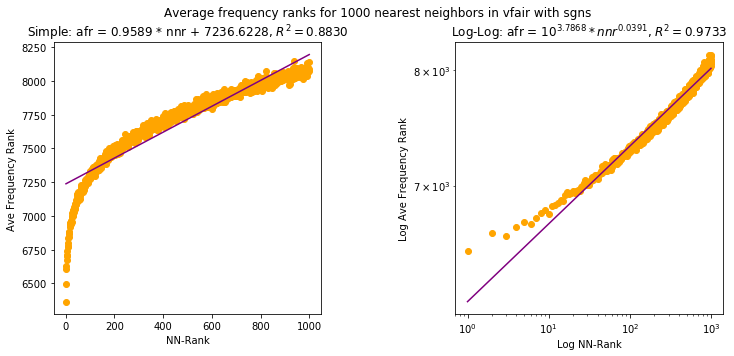Dot products of sims with mean by frequency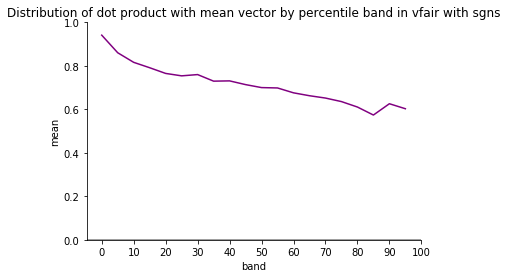Dimension values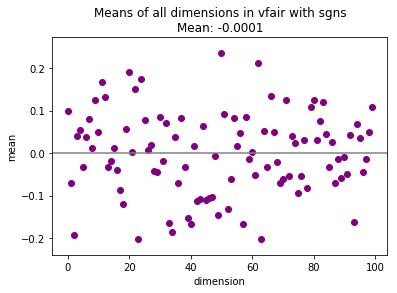Finally, hubs need a combination of table and visualization: the particular hubs in a table and their distribution in a chart.

In :
smplr = vfair_all['sampler']
vname = 'sgns'
vs = vfair_all[vname]
name = 'vfair with %s' % vname
tests = ['hubs','hubp']
testfs(name,smplr,vs,tests=tests)

Summary of possible frequency effects for vfair with sgns


Top 10 Hubs
overall mean = 1000.0000
overall std = 741.2560
word percentile # stds
knack 0 4.965896
weighed 1 4.772980
dolly 1 4.771631
rigidly 0 4.740602
contentedly 1 4.725763
canal 1 4.582762
sailor 1 4.553083
tops 1 4.507215
boiled 1 4.473488
bravely 1 4.439762
Hub percentiles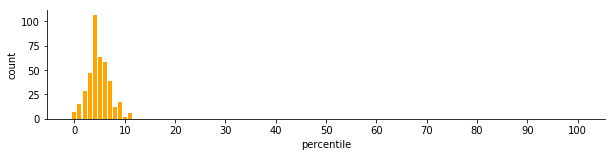121/400 = 0.302 of the hubs were in the same band as comparison



## Comparions of sgns and cbow¶

By having this suite of tests, it is also easy to compare different embeddings, whether using different corpora or different methods. In this section, we'll use the tests to compare the other word2vec method, cbow, with sgns shown above.

First, the table.

In :
vfair_all['cbow'] = Setup.make_vecs('cbow', vfair_all['sampler'].sents, 1,5,100,init_sims=True) #window=5, dims=100, min_count=1
heartd_all['cbow'] = Setup.make_vecs('cbow', heartd_all['sampler'].sents, 1,5,100,init_sims=True) #window=5, dims=100, min_count=1

In :
smplr = vfair_all['sampler']
vname = 'cbow'
vs = vfair_all[vname]
name = 'vfair with %s' % vname
tests = ['vfreq','sksim','stfreq','strank','strecip']
testfs(name,smplr,vs,tests=tests)

Testing Vectors ∝ freqs
Testing Vectors ∝ non-v. low freqs
Testing Vectors ∝ non-low freqs
Testing Skewed sims
Testing Stratification of freq
Testing Stratification of rank
Testing Stratification of recip

Summary of possible frequency effects for vfair with cbow
Aspect Result Details
Vectors ∝ freqs weak percentiles 0-100, R2 = 0.0337
Vectors ∝ non-v. low freqsweak percentiles 1-100, R2 = 0.1134
Vectors ∝ non-low freqs moderate percentiles 5-100, R2 = 0.5287
Skewed sims moderate mean = 0.7467, variance = 0.0700
Stratification of freq moderate, inverse R2 = 0.3957
Regression coefficient: c = -0.0009
Stratification of rank moderate
ref: weak, direct
comp: moderate, inverse
R2 = 0.5483
Regression coefficients:
cref = -0.0002, ccomp = 0.0034
ref. Pearson = -0.0431
comp. Pearson = 0.7392
Stratification of recip strong, inverse Pearson = 0.7506




What see from the table is that cbow has very different properties from sgns. In particular, it shows only weak to moderate frequency effects, unlike sgns which showed mostly strong frequency effects.

Next the charts. The results for cbow here are also different from those for sgns. The power law is much less clear, and theres is not a consistent pattern of the dot product with the mean, unlike there is with sgns. The only thing in common is the lack of frequency effect for the dimension values.

In :
smplr = vfair_all['sampler']
vname = 'cbow'
vs = vfair_all[vname]
name = 'vfair with %s' % vname
tests = ['vpower','dpmean','dims']
testfs(name,smplr,vs,tests=tests)

Summary of possible frequency effects for vfair with cbow


Nearest neighbor frequency power law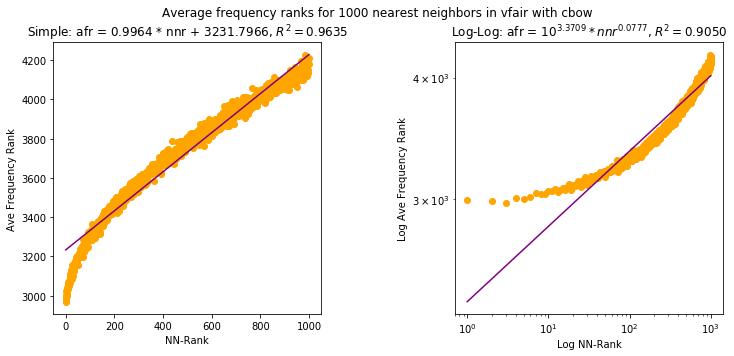Dot products of sims with mean by frequency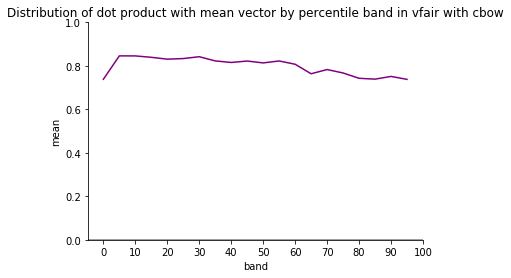Dimension values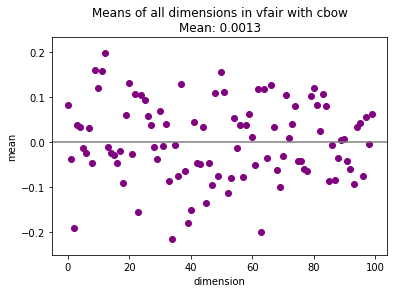Finally, the hubs. The main thing to notice is that the hubs with cbow are a bit more distributed across the percentiles, though they are still concentrated in the 0-10 percentile band.

In :
smplr = vfair_all['sampler']
vname = 'cbow'
vs = vfair_all[vname]
name = 'vfair with %s' % vname
tests = ['hubs','hubp']
testfs(name,smplr,vs,tests=tests)

Summary of possible frequency effects for vfair with cbow


Top 10 Hubs
overall mean = 1000.0000
overall std = 1222.6593
word percentile # stds
selfishness 5 3.665780
doubly 2 3.653512
five 22 3.640426
napoleon 7 3.568451
busy 6 3.562726
prince 15 3.539007
dismal 8 3.537371
hot 7 3.525921
rage 9 3.525103
horse 15 3.512835
Hub percentiles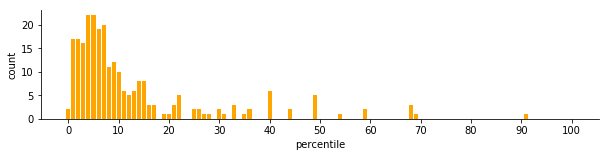57/253 = 0.225 of the hubs were in the same band as comparison



Given that cbow is so different from sgns with vfair, let's see how they compare on Heart of Darkness.

First the tables. For heartd, the two word2vec approaches are similar, both showing only moderate to weak effects, unlike sgns for vfair. Note too that the direction of the correlations are different between vfair and heartd.

vfairheartd
Frequencyinversedirect
Rank referencedirectdirect
Rank comparitorinversedirect
Reciprocityinverseinverse
In :
smplr = heartd_all['sampler']

tests = ['vfreq','sksim','stfreq','strank','strecip']

vname = 'sgns'
name = 'heartd with %s' % vname
vs = heartd_all[vname]
testfs(name,smplr,vs,tests=tests)

vname = 'cbow'
name = 'heartd with %s' % vname
vs = heartd_all[vname]
testfs(name,smplr,vs,tests=tests)

Testing Vectors ∝ freqs
Testing Vectors ∝ non-v. low freqs
Testing Vectors ∝ non-low freqs
Testing Skewed sims
Testing Stratification of freq
Testing Stratification of rank
Testing Stratification of recip

Summary of possible frequency effects for heartd with sgns
Aspect Result Details
Vectors ∝ freqs weak percentiles 0-100, R2 = 0.0566
Vectors ∝ non-v. low freqsweak percentiles 1-100, R2 = 0.0502
Vectors ∝ non-low freqs moderate percentiles 5-100, R2 = 0.4366
Skewed sims moderate mean = 0.2997, variance = 0.2164
Stratification of freq weak, direct R2 = 0.1811
Regression coefficient: c = 0.0016
Stratification of rank moderate
ref: weak, direct
comp: weak, direct
R2 = 0.2598
Regression coefficients:
cref = -0.0009, ccomp = -0.0021
ref. Pearson = -0.1910
comp. Pearson = -0.4726
Stratification of recip weak, inverse Pearson = 0.3303


Testing Vectors ∝ freqs
Testing Vectors ∝ non-v. low freqs
Testing Vectors ∝ non-low freqs
Testing Skewed sims
Testing Stratification of freq
Testing Stratification of rank
Testing Stratification of recip

Summary of possible frequency effects for heartd with cbow
Aspect Result Details
Vectors ∝ freqs weak percentiles 0-100, R2 = 0.0963
Vectors ∝ non-v. low freqsweak percentiles 1-100, R2 = 0.0894
Vectors ∝ non-low freqs weak percentiles 5-100, R2 = 0.1010
Skewed sims moderate mean = 0.2140, variance = 0.1251
Stratification of freq weak, direct R2 = 0.2201
Regression coefficient: c = 0.0020
Stratification of rank moderate
ref: weak, direct
comp: moderate, direct
R2 = 0.3194
Regression coefficients:
cref = -0.0008, ccomp = -0.0024
ref. Pearson = -0.1845
comp. Pearson = -0.5342
Stratification of recip weak, inverse Pearson = 0.4100




Next up: the charts. Once again, the two approachs are somewhat similar. While they both lack a clear power-law relation, with sgns the data seems linear where it does not with cbow.

In :
smplr = heartd_all['sampler']

tests = ['vpower','dpmean','dims']

vname = 'sgns'
name = 'heartd with %s' % vname
vs = heartd_all[vname]
testfs(name,smplr,vs,tests=tests)

vname = 'cbow'
name = 'heartd with %s' % vname
vs = heartd_all[vname]
testfs(name,smplr,vs,tests=tests)

Summary of possible frequency effects for heartd with sgns


Nearest neighbor frequency power law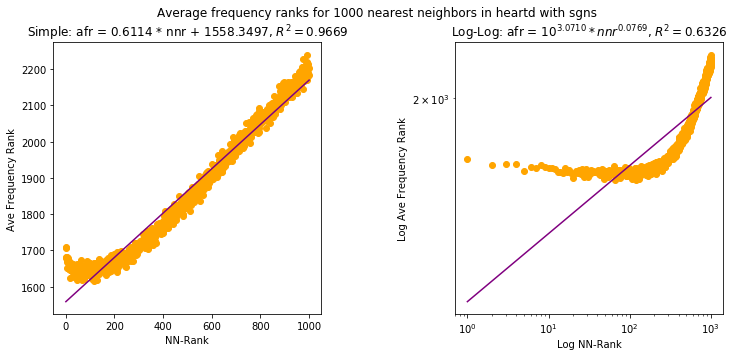Dot products of sims with mean by frequency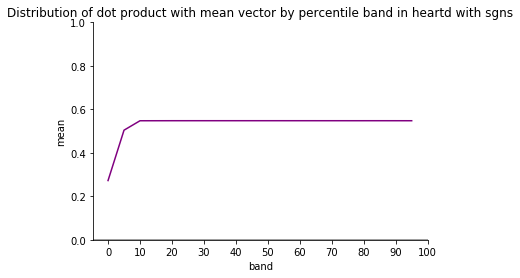Dimension values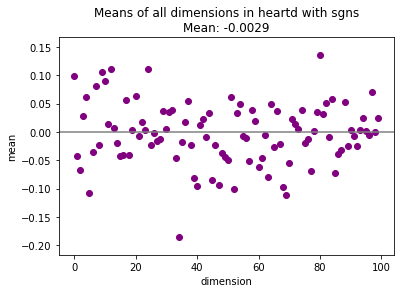Summary of possible frequency effects for heartd with cbow


Nearest neighbor frequency power law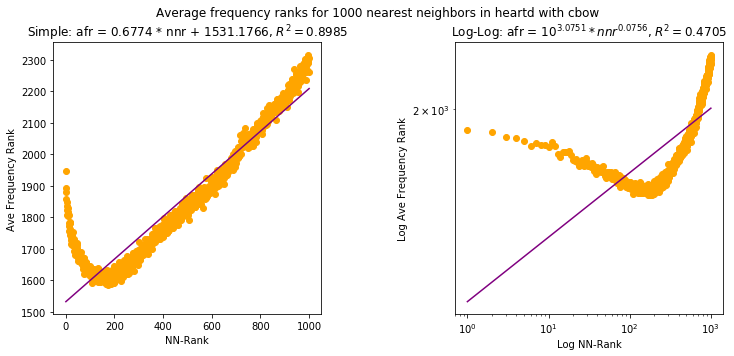Dot products of sims with mean by frequency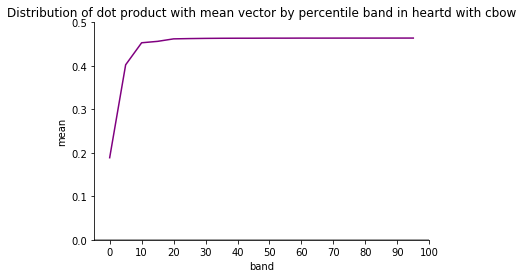Dimension values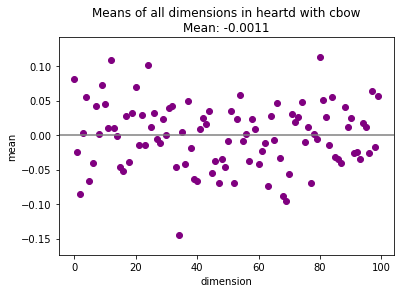And finally, the hubs, where the approaches are again similar.

In :
smplr = heartd_all['sampler']

tests = ['hubs','hubp']

vname = 'sgns'
name = 'heartd with %s' % vname
vs = heartd_all[vname]
testfs(name,smplr,vs,tests=tests)

vname = 'cbow'
name = 'heartd with %s' % vname
vs = heartd_all[vname]
testfs(name,smplr,vs,tests=tests)

Summary of possible frequency effects for heartd with sgns


Top 10 Hubs
overall mean = 1000.0000
overall std = 923.6922
word percentile # stds
comes 5 2.239924
candle 4 2.237758
fresh 2 2.237758
live 9 2.232345
quiet 9 2.231263
continent 3 2.230180
sight 8 2.229097
street 3 2.228015
sky 8 2.228015
woman 5 2.226932
Hub percentiles
Didn't find hubs for percentile bands at the threshold of 2 standard deviations


Summary of possible frequency effects for heartd with cbow


Top 10 Hubs
overall mean = 1000.0000
overall std = 916.6305
word percentile # stds
board 6 2.298636
english 9 2.295363
straight 8 2.293181
special 2 2.293181
wreck 2 2.292090
street 3 2.290999
expression 10 2.290999
comes 5 2.289908
cold 4 2.288818
opened 8 2.287727
Hub percentiles
Didn't find hubs for percentile bands at the threshold of 2 standard deviations



What we have seen is that sgns and cbow differ dramatically on the larger corpus vfair but are very similar on the smaller corpus heartd. There are also differences between vfair and cbow, for example in the trends for the dot product. The summary tests make these differences easy to see.

In the first set of posts we consistently saw differences between vfair and heartd, and we see differences again here with cbow. This raises the question of how much corpus size affects the frequency effects. In the next post, we'll look at some large corpora.

## Reference¶

 Tomas Mikolov, Ilya Sutskever, Kai Chen, Gregory S. Corrado, and Jeffrey Dean. 2013. Distributed representations of words and phrases and their composi- tionality. In Advances in Neural Information Processing Systems 26: 27th Annual Conference on Neural Information Processing Systems 2013. Proceedings of a meeting held December 5-8, 2013, Lake Tahoe, Nevada, United States. 3111–3119.